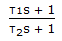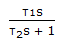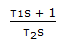# Chemical Engineering - Process Control and Instrumentation

### Exercise :: Process Control and Instrumentation - Section 3

31.

The response of two tanks of same size and resistance in series is

 A. under damped B. critically damped C. over damped D. none of the above

Explanation:

No answer description available for this question. Let us discuss.

32.

The frequency response of a dynamic element shows a constant magnitude ratio at all frequencies. The element exhibits a negative phase shift at all frequencies. The absolute value of the phase shift increases linearly with frequency. The element has the transfer function

 A. e-τs B.C.D.Explanation:

No answer description available for this question. Let us discuss.

33.

The amplitude ratio for the sinusoidal response of __________ is < 1.

 A. transportation lag B. first order system C. second order system D. none of these

Explanation:

No answer description available for this question. Let us discuss.

34.

Which of the following is a 'contact' pyrometer ?

 A. resistance pyrometer B. optical pyrometer C. radiation pyrometer D. infra red pyrometer

Explanation:

No answer description available for this question. Let us discuss.

35.

Which of the following is not a speed measuring instrument ?

 A. Psychrometer B. Stroboscope C. Tachometer D. All (a), (b) and (c)